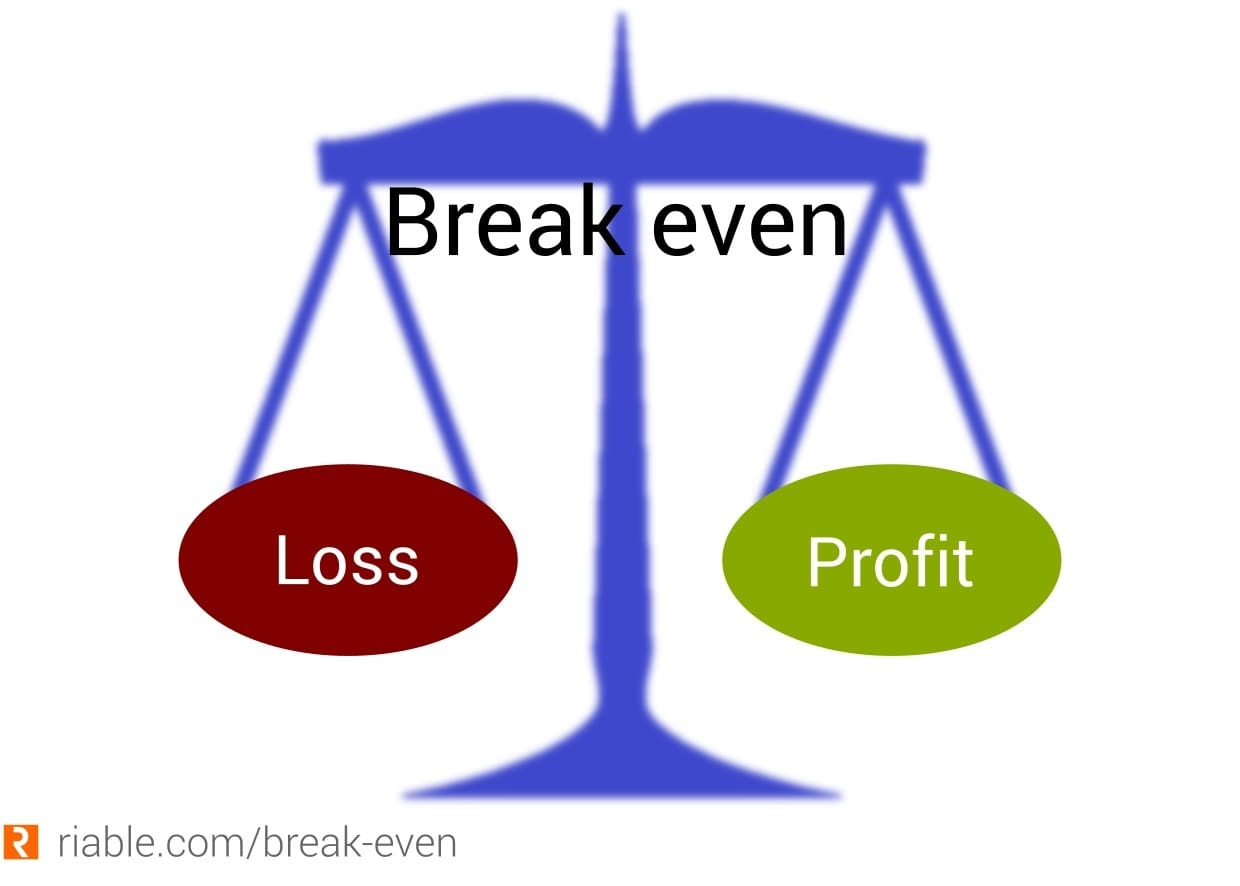## Railway Accounts Department Examinations

Showing posts with label Fixed Cost. Show all posts
Showing posts with label Fixed Cost. Show all posts

## Saturday, January 28, 2023

### Fixed Cost and its importance in BEPFixed Cost & its importance in BEP

• Meaning of Fixed Cost :  A cost does not change with an increase or decrease in the Goods produced.

• In General, companies can have two types of costs, i.e., Fixed Costs and Variable Costs.

• Also called as Indirect cost or Overhead costs.

• Examples:  Lease Rentals, Salaries, Insurance, Taxes, Interest expense, Depreciation etc.

• All Sunk costs are Fixed Costs.  But, all fixed costs are not sunk costs.

• Sunk cost: Money that has already been spent and which cannot be recovered.  Examples are Machinery Cost, Lease expense, etc.

• A fixed cost per unit is always variable; Whereas Variable cost per unit is always fixed.

• Segregation of Total Costs into fixed Costs and Variable costs helps the Management to decide the scale of Production and Breakeven analysis.

• Example: A company Produces Pens.  Their fixed costs are Rs. 10000 and Variable costs are Rs. 10 per Pen. Selling price per pen is Rs. 120.  Find the Total cost for 100 Pens and 200 Pens and Profit on two options.

100 Units

 Costs No of Pens Rate per Unit Total Fixed 100 100 10000 Variable Costs 100 10 1000 Total costs 100 110 11000 Selling Price 100 120 12000 Profit 100 10 1000

200 Units:

Costs

No of Pens

Rate per Unit

Fixed

200

50

10000

Variable Costs

200

10

2000

Total

200

60

12000

Selling price

200

120

24000

Profit

200

60

12000

• From the above, the Fixed Cost per Unit is changed from Rs. 100 to Rs.50 when Production was increased from 100 units to 200 Units.  Whereas, Variable cost remained fixed though production was increased from 100 units to 200 units.

So, when change in the Production:

• Fixed cost per unit is variable  (though Total Fixed cost is fixed)

• Variable cost per unit is fixed. (though Total Variable cost is variable)

• The segregation of Total costs into Fixed Costs and Variable costs helps the firms to analyze the breakeven analysis. (Where there is no profit, no loss) and increase their profit capacity.

BEP –Break Even Point = Fixed Costs / Sales Price per Unit – Variable Cost per unit

• In the above example 100 units production level, BEP is 10000/120 – 10 = 91 Units.

• That means at the point of 91 units, there is no profit or no loss.

At 91 units

 Costs No of Pens Rate per Unit Fixed 91 109.90 10000 Variable Costs 91 10 910 Total 91 119.90 10911 Selling price 91 120 10920 Profit 91 0.10 9

Profit Rs.9 is almost negligible.  Hence at the production of 91 units, there is no profit, and there is no loss.   So Break Even Point (BEP) is 91 units

Key Points for MCQ

1. BEP stands for Break Even Point

1. Fixed cost - other names are Overhead cost or Indirect Cost

1. All Sunk costs are Fixed Costs.  But, all fixed costs are not sunk costs.

1. Sunk cost: Money that has already been spent and which cannot be recovered.  Examples are Machinery Cost, Lease expense, etc.

1. A fixed cost per unit is always variable

1. Whereas Variable cost per unit is always fixed

1. BEP formula = Fixed Costs / Sales Price per Unit – Variable Cost per unit

###